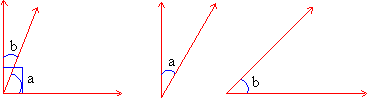Home MonkeyNotes Printable Notes Digital Library Study Guides Message Boards Study Smart Parents Tips College Planning Test Prep Fun Zone Help / FAQ How to Cite New Title Request

 1.4 Some special angles Complementary angles : If the sum of two angles equals 900 the two angles are called complementary. Complementary angles thus add up to a right angle. Complementary angles are of two types. If they have one side in common they are called adjacent complementary angles ( figure 1.20 a ). If no side is common then they are called non-adjacent complementary angles ( figure 1.20 b ).Figure 1.20 a                          Figure 1.20 b Since the measures of complementary angles always sum up to 900 if the measure of one angle is known that of the complement can be found easily. In figure 1.20 b Ð a and Ð b are complementary. Also it is known that m Ð a = 300. m Ð a + m Ð b = 900     300 + m Ð b = 900               m Ð b = 900 - 300               m Ð b = 600 Theorem : If two angles are complementary to a third angle, then they are equal to each other. Proof : Ð a and Ð b are both complementary to Ð c. \ m Ð a + m Ð c = 900 and also m Ð b + m Ð c = 900 \ m Ð a + m Ð c = m Ð b + m Ð c OR m Ð a = m Ð b Index Introduction 1.1 Points, Lines and Planes 1.2 Line Segment 1.3 Rays and Angles 1.4 Some Special Angles 1.5 Angles made by a Transversal 1.6 Transversal Across Two Parallel Lines 1.7 Conditions for Parallelism Chapter 2
 Search: All Products Books Popular Music Classical Music Video DVD Toys & Games Electronics Software Tools & Hardware Outdoor Living Kitchen & Housewares Camera & Photo Cell Phones Keywords: